# Capacitor Color Codes:

### Introduction

Capacitance of a capacitor is the ability of a capacitor to store maximum charge on its plates. The capacitance of a capacitor is measured in the units of Farads. Generally the capacitance values, working voltage and tolerance values are indicated on the body of a capacitor.
But sometimes it is difficult to identify these capacitance and voltage values on the capacitor body in the case of decimal values. It also causes misreading of the actual capacitance and voltage values. So , a technique was used to identify the capacitance values by using the letters like p (pico) and n (nano) instead of decimal values (such as 200 k=200*1000 pF=200 nF and 47 n=47 nF,  n47=0.47nF etc).
So to avoid these problems a color scheme was introduced for capacitors like resistors. This colour scheme for capacitors is usually called as capacitor color coding. In this scheme each color of a capacitor indicates a specific capacitance value. Using this color scheme we can easily identify the capacitance values, voltages and tolerance values of any capacitor. These color schemes ;color coding and colors assigned for values are explained in below.

### Table:Capacitor Color Code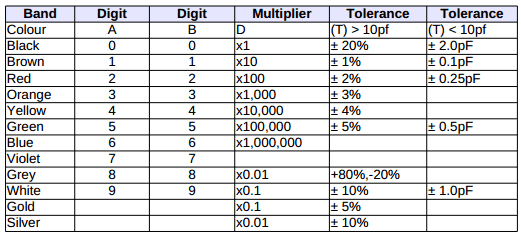### Table :Capacitor Voltage Color Code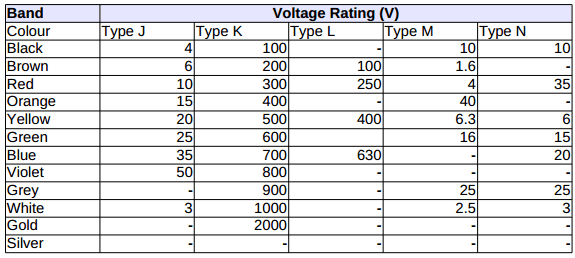### Capacitor Voltage Reference

The capacitor has the capacitance value, voltage , tolerance and manufacturer numbers on the body of a capacitor. Some voltage values are used as references for working voltages of a capacitor. In this representation we see some letters or characters like J, K, N, M etc. Now let us the meaning of those letters which are used on the body of a capacitor.
J-Type => Dipped Tantalum type capacitors
K-Type => Mica type capacitors
L-Type +> Polyester (or) Polystyrene type capacitors
M-Type => Electrolytic 4 Band type capacitors
N-Type => Electrolytic 3 Band type capacitors

### Metalized Polyester CapacitorFigure 1.Metalized Polyester Capacitors with color codes
The capacitors which are shown in the above figure are metalized polyester capacitors with color codes. In this each color represents a specific parameter for capacitance values, tolerance and working voltages. All the above capacitors have different capacitance and tolerance values.These values can be understood by the color code table which is given at the side of capacitors in the above figure.

### Disc & Ceramic Capacitor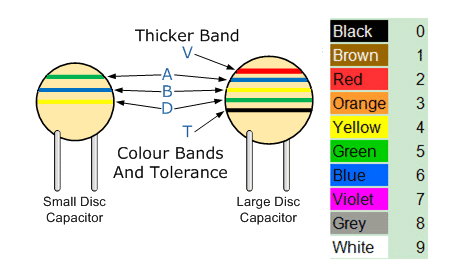Figure 2. Disc capacitors with colour codes
The above figure shows the Disc and Ceramic capacitors with color codes. These colour codes are used from many years for non-polarized capacitors like disc and ceramic capacitors. But it is difficult to identify the values in the case of old capacitors. So these old capacitors are now replaced with new numbers.
In three digit number representation , the third number represents number of zeros, such as 471=470pF, 101=100pF. In the case of two digit number representation tolerance is also identified. In two number representation disc or film capacitors have the capacitance value usually in pico-Farads, for example 47=47pF, 20=20pF. In the above figure we observed the capacitance values and tolerance for small disc or large disc capacitors can be calculated by using color code which is shown at the side of capacitors.

### Capacitor Tolerance Letter Codes Table

Capacitor has numbers and letters on its body to identify the capacitance values and tolerance values respectively.Letters for the specific tolerance value representation is shown in the below table. Now we will see one example to understand this concept below.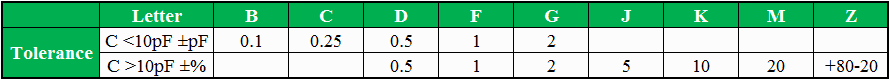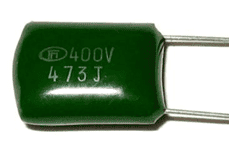The capacitor which is shown in the above figure has 473 J code on its body. Here 4 is first digit, 7 is second digit and 3 is the number of zeros i.e. the capacitance value is 47*1000pF=47000pF=47nF=0.047uF. Here letter ‘J’ denotes the tolerance of a capacitor, referring to above table , tolerance of this capacitor is +/-5%. In this way by just using numbers and letters on the body of a capacitor, we can easily determine the capacitance values and tolerances of capacitors.

### Table:Capacitor Letter Codes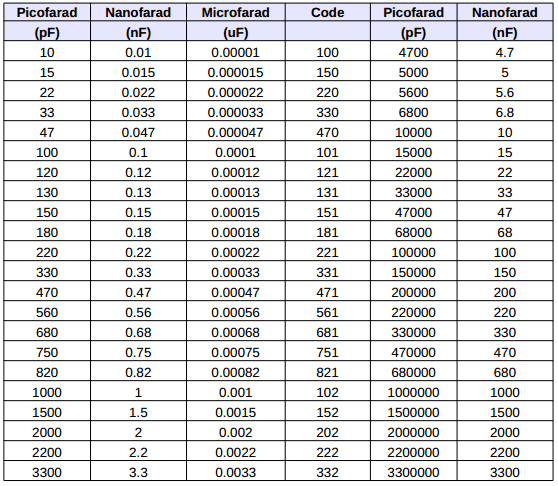The capacitance values of capacitor are  measured in pico-Farads, nano-Farads, or micro-Farads. The relation between these values for different letter codes is shown in the above table. From this table we clearly can understand about the units of capacitance. The basic relation between them is 1uF=1000nf=1000000pF
REFERENCE // Electronics Hub